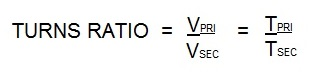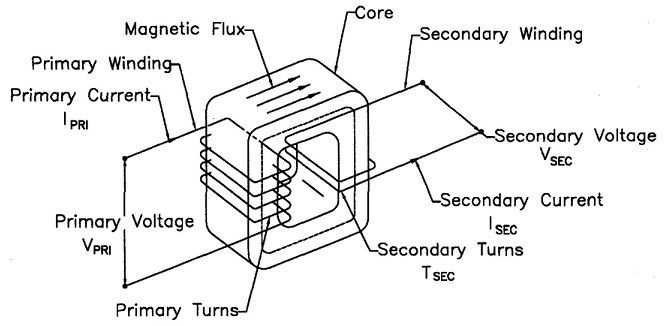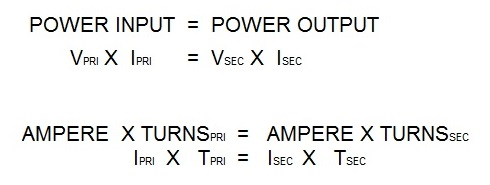# Spot Welding

To answer this question I will demonstrate the calculation of the turns ratio for a AC 16 KVA resistance welding transformer.

The turns ratio for all resistance welding transformers is calculated in the same manner no matter the KVA size. Turns ratio is the ratio of the number of coil turns in the primary vs the secondary.In AC transformers, there are many turns in the primary. The secondary has one turn. If there are 50 turns then the ratio is 50/1. Therefor the primary voltage will drop from 220V and 100 amp input to:

220/50 = 4.4 volts in the secondary. The equations for this are:Vpri = Voltage on the primary                          Tpri = Turns in the primary coil
Vsec= Voltage on the secondary                     Tsec = Turns in the secondary coilPower into the transformer will equal power out of the transformerIsec = Secondary amperage                 Amperage Ipri = Primary amperage

In the secondary using the above formula:
If the Ipri = 100 amperes
If the turns ratio = 50/1
Isec = (Ipri X Tpri)/ Tsec
Isec = 100 x 50/1
Isec = 5000 amperes

There are several additional articles written on turns ratio available in the “HOW TO RESISTANCE WELD” blog.

Reference: RWMA Manual Chapter 19

Yes, there is a basic difference between the MFDC and AC transformers. MFDC transformers normally operate at 1000 Hz frequency and AC transformers operate at either 60 or 50 Hz depending upon location (N. America or Europe, etc).

Soldering by definition is a process performed below 450 deg C.  A review of the literature found that resistance methods are sometime used for soldering when open flames are not practical or permitted.  Automation is possible but manual operation is common. The electrodes are generally highly resistive as in graphite, carbon or tungsten. The carbon or graphite may be copper clad. The tungsten could be a copper tungsten RWAMA Class 11.

This process is using relatively hot electrodes to generate heat to help melt the solder.

Reference: ASM Handbook Vol. 6 Welding, Brazing, Soldering

This question has been answered already in another article in this blog:

IF COOLING WATER IS GROUNDED TO THE MACHINE AND PLANT WHY DOES CURRENT CONTINUE TO FLOW THROUGH THE ELECTRODES IN AC AND DC RESISTANCE WELDING

That article stated that the normal low voltage of 4-6 volts cannot overcome the resistivity of the water. Since the resistivity of the copper conductors is very low. The current flows preferentially (thousands of amperes) through the conductors and electrodes and a negligible amount flows through the water.

This assumes that the water is maintained properly as specified by:

AWS STANDARD J1.2 “GUIDE TO INSTALLATION AND MAINTANANCE OF RESISTANCE WELDING MACHINES”

Reference: AWS J1.2M:2016
Resistance Welding Manual, 4th Edition

In a normal spot weld set up, the pair of electrodes would be matched in alloy and face diameter in order to generate equal heat to the part from each side.  Certain applications due to material or part design dictate a change from this balanced approach.  Two electrodes of different alloys or design are frequently used to balance the heat and keep the nugget formation at the desired location. This is call “Heat Balancing”.

Another article in the blog describes “Heat Balancing”

“WHAT IS HEAT BALANCING”

### Have a Question?

Do you have a question that is not covered in our knowledgebase? Do you have questions regarding the above article? Click here to ask the professor.